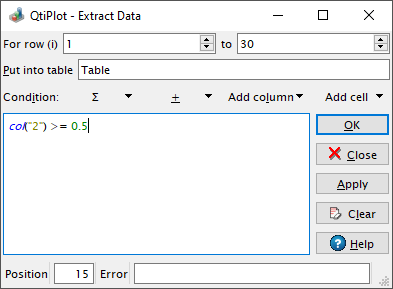# Extract Data

This dialog is activated with the Extract Data... command from the Table menu. It opens a dialog which allows you to define a set of conditions that are used to filter the data in the currently active table. When a condition has been defined, Applying the condition will create a new table into which all rows that meet the condition will be copied. The original table is unchanged. For example, the condition col("1") > 0.5 will generate a new table that contains all the rows from the active table which have a value greater than 0.5 in column 1.

Figure 5-179. The Extract Data... dialog.The available mathematical functions can be viewed with the help of the function selector button which displays the capital greek letter sigma ∑. By pressing this button a dropdown menu of intrinsic functions grouped by the first letter of their name will be shown. Selecting a function name will copy it into the function definition pane. Details of these functions and supported mathematical operators are listed in the muParser section in the chapter on Mathematical Expressions and Scripting. muParser is the only scripting language that can be used in this dialog.

The special function, col(c), is used to access the values of column c, where c is the column's number (as in col(2)) or its name in double quotes (as in col("time")). You can also obtain values from other tables using the tablecol(t,c) function, where t is the table's name in double quotes and c is the column's number or its name in double quotes (example: tablecol("Table1","time")).

The variables i and j can be used to access the current row and column numbers. Similarly, sr and er represent the selected starting and ending row, respectively.

The filtering condition is built with the common operators +, -, *, /, %, and ^ (for addition, subtraction, multiplication, division, modulus operator and exponentiation, respectively) and the logical operators: >, >=, <, <=, !=, ==. These operators can be added by pressing the + button and choosing the operator needed from the dropdown menu.